# Excel MID function to extract text from the middle of a string

MID is one of the Text functions that Microsoft Excel provides for manipulating text strings. At the most basic level, it is used to extract a substring from the middle of the text string. In this tutorial, we will discuss the syntax and specificities of the Excel MID function, and then you will learn a few creative uses to accomplish challenging tasks.

## Excel MID function - syntax and basic uses

Generally speaking, the MID function in Excel is designed to pull a substring from the middle of the original text string. Technically speaking, the MID function returns the specified number of characters starting at the position you specify.

The Excel MID function has the following arguments:

MID(text, start_num, num_chars)

Where:

• Text is the original text string.
• Start_num is the position of the first character that you want to extract.
• Num_chars is the number of characters to extract.

All 3 arguments are required.

For example, to pull 7 characters from the text string in A2, starting with the 8th character, use this formula:

`=MID(A2,8, 7)`

The result might look something similar to this: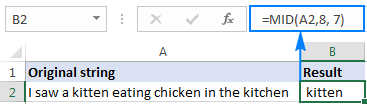### 5 things you should know about Excel MID function

As you have just seen, there's no rocket science in using the MID function in Excel. And remembering the following simple facts will keep you safe from most common errors.

1. The MID function always returns a text string, even if the extracted substring contains only digits. This may be critical if you wish to use the result of your Mid formula within other calculations. To convert an output into a number, use MID in combination with the VALUE function as shown in this example.
2. If start_num is greater than the overall length of the original text, an Excel Mid formula returns an empty string ("").
1. If start_num is less than 1, a Mid formula returns the #VALUE! error.
2. If num_chars is less than 0 (negative number), a Mid formula returns the #VALUE! error. If num_chars is equal to 0, it outputs an empty string (blank cell).
3. If the sum of start_num and num_chars exceeds the total length of the original string, the Excel MID function returns a substring starting from start_num and up to the last character.

## Excel MID function - formula examples

When dealing with real-life tasks in Excel, you will most often need to use MID in combination with other functions as demonstrated in the following examples.

### How to extract first and last name

If you've had a chance to read our recent tutorials, you already know how to pull the first name using the LEFT function and get the last name with the RIGHT function. But as is often the case in Excel, the same thing can be done in a variety of ways.

#### MID formula to get the first name

Assuming the full name is in cell A2, first and last names separated with a space character, you can pull the first name using this formula:

`=MID(A2,1,SEARCH(" ",A2)-1)`

The SEARCH function is used to scan the original string for the space character (" ") and return its position, from which you subtract 1 to avoid trailing spaces. And then, you use the MID function to return a substring beginning with the fist character and up to the character preceding the space, thus fetching the first name.

#### MID formula to get the last name

To extract the last name from A2, use this formula:

`=TRIM(MID(A2,SEARCH(" ",A2),LEN(A2)))`

Again, you use the SEARCH function to determine the starting position (a space). There is no need for us to calculate the end position exactly (as you remember, if start_num and num_chars combined is bigger than the total string length, all remaining characters are returned). So, in the num_chars argument, you simply supply the total length of the original string returned by the LEN function. Instead of LEN, you can put a number that represents the longest surname you expect to find, for example 100. Finally, the TRIM function removes extra spaces, and you get the following result: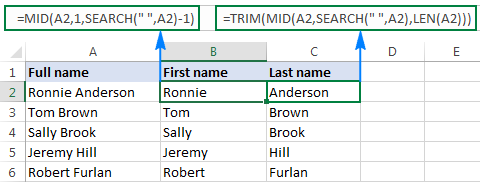Tip. To extract the first and last word from a string with a simpler formula, you can use the custom ExtractWord function.

### How to get substring between 2 delimiters

Taking the previous example further, if besides first and last names cell A2 also contains a middle name, how do you extract it?

Technically, the task boils down to working out the positions of two spaces in the original string, and you can have it done in this way:

• Like in the previous example, use the SEARCH function to determine the position of the first space (" "), to which you add 1 because you want to start with the character that follows the space. Thus, you get the start_num argument of your Mid formula: SEARCH(" ",A2)+1
• Next, get the position of the 2nd space character by using nested Search functions that instruct Excel to start searching from the 2nd occurrence of the space character: SEARCH(" ",A2,SEARCH(" ",A2)+1)

To find out the number of characters to return, subtract the position of the 1st space from the position of the 2nd space, and then subtract 1 from the result since you don't want any extra spaces in the resulting substring. Thus, you have the num_chars argument: SEARCH (" ", A2, SEARCH (" ",A2)+1) - SEARCH (" ",A2)

With all the arguments put together, here comes the Excel Mid formula to extract a substring between 2 space characters:

=MID(A2, SEARCH(" ",A2)+1, SEARCH (" ", A2, SEARCH (" ",A2)+1) - SEARCH (" ",A2)-1)

The following screenshot shows the result: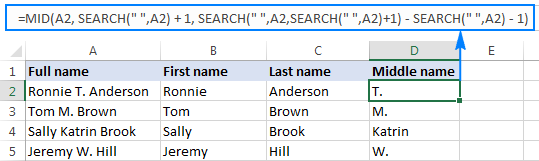In a similar manner, you can extract a substring between any other delimiters:

MID(string, SEARCH(delimiter, string)+1, SEARCH (delimiter, string, SEARCH (delimiter, string)+1) - SEARCH (delimiter, string)-1)

For example, to pull a substring that is separated by a comma and a space, use this formula:

=MID(A2,SEARCH(", ",A2)+1,SEARCH(", ",A2,SEARCH(", ",A2)+1)-SEARCH(", ",A2)-1)

In the following screenshot, this formula is used to extract the state, and it does the job perfectly: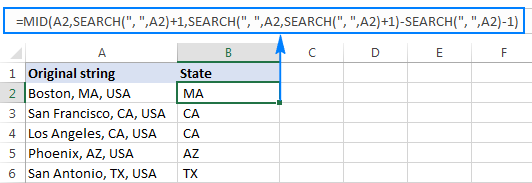### How to extract Nth word from a text string

This example demonstrates an inventive use of a complex Mid formula in Excel, which includes 5 different functions:

• LEN - to get the total string length.
• REPT - repeat a specific character a given number of times.
• SUBSTITUTE - replace one character with another.
• MID - extract a substring.
• TRIM - remove extra spaces.

The generic formula is as follows:

TRIM(MID(SUBSTITUTE(string," ",REPT(" ",LEN(string))), (N-1)*LEN(string)+1, LEN(string)))

Where:

• String is the original text string from which you want to extract the desired word.
• N is the number of word to be extracted.

For instance, to pull the 2nd word from the string in A2, use this formula:

`=TRIM(MID(SUBSTITUTE(A2," ",REPT(" ",LEN(A2))), (2-1)*LEN(A2)+1, LEN(A2)))`

Or, you can input the number of the word to extract (N) in some cell and reference that cell in your formula, like shown in the screenshot below: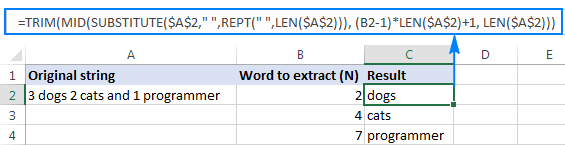#### How this formula works

In essence, the formula wraps each word in the original string with many spaces, finds the desired "spaces-word-spaces" block, extracts it, and then removes extra spaces. To be more specific, the formula works with the following logic:

• The SUBSTITUTE and REPT functions replace each space in the string with multiple spaces. The number of additional spaces is equal to the total length of the original string returned by LEN: SUBSTITUTE(A2," ",REPT(" ",LEN(A2)))

You can think of an intermediate result as of "asteroids" of words drifting in space, like this: spaces-word1-spaces-word2-spaces-word3-… This "spacious" string is supplied to the text argument of our Mid formula.

• Next, you work out the starting position of the substring of interest (start_num argument) using the following equation: (N-1)*LEN(A1)+1. This calculation returns either the position of the first character of the desired word or, more often, the position of some space character in the preceding space separation.
• The number of characters to extract (num_chars argument) is the easiest part - you simply take the overall length of the original string: LEN(A2). At this point, you are left with spaces-desired word-spaces substring.
• Finally, the TRIM function gets rid of leading and trailing spaces.

The above formula works fine in most situations. However, if there happen to be 2 or more consecutive spaces between words, it yields wrong results. To fix this, nest another TRIM function inside SUBSTITUTE to remove excess in-between spaces except for a single space character between words, like this:

`=TRIM(MID(SUBSTITUTE(TRIM(A2)," ",REPT(" ",LEN(A2))), (B2-1)*LEN(A2)+1, LEN(A2)))`

The following screenshot demonstrates the improved formula in action: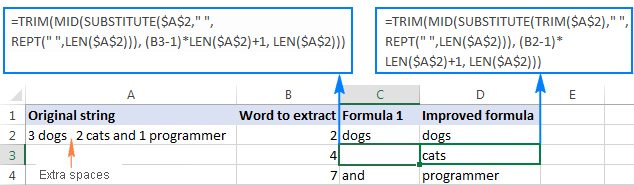If your source strings contain multiple spaces between words as well as very big and very small words, additionally embed a TRIM function into each LEN, just to keep you on the safe side:

`=TRIM(MID(SUBSTITUTE(TRIM(A2)," ",REPT(" ",LEN(TRIM(A2)))), (B2-1)*LEN(TRIM(A2))+1, LEN(TRIM(A2))))`

I agree that this formula looks a bit cumbersome, but it impeccably handles all kinds of strings.

Tip. See how to extract any Nth word from text using a more compact and straightforward formula.

### How to extract a word containing a specific character(s)

This example shows another non-trivial Excel Mid formula that pulls a word containing a specific character(s) from anywhere in the original text string:

TRIM(MID(SUBSTITUTE(string," ",REPT(" ",99)),MAX(1,FIND(char,SUBSTITUTE(string," ",REPT(" ",99)))-50),99))

Assuming the original text is in cell A2, and you are looking to get a substring containing the "\$" character (the price), the formula takes the following shape:

`=TRIM(MID(SUBSTITUTE(A2," ",REPT(" ",99)),MAX(1,FIND("\$",SUBSTITUTE(A2," ",REPT(" ",99)))-50),99))`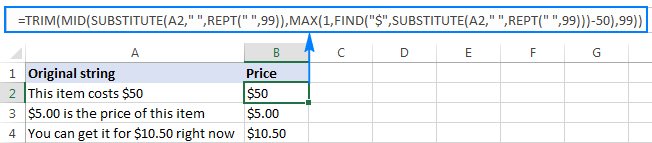In a similar fashion, you can extract email addresses (based on the "@" char), web-site names (based on "www"), and so on.

#### How this formula works

Like in the previous example, the SUBSTITUTE and REPT functions turn every single space in the original text string into multiple spaces, more precisely, 99 spaces.

The FIND function locates the position of the desired character (\$ in this example), from which you subtract 50. This takes you 50 characters back and puts somewhere in the middle of the 99-spaces block that precedes the substring containing the specified character.

The MAX function is used to handle the situation when the desired substring appears in the beginning of the original text string. In this case, the result of FIND()-50 will be a negative number, and MAX(1, FIND()-50) replaces it with 1.

From that starting point, the MID function collects the next 99 characters and returns the substring of interest surrounded by lots of spaces, like this: spaces-substring-spaces. As usual, the TRIM function helps you eliminate extra spaces.

Tip. If the substring to be extracted is very big, replace 99 and 50 with bigger numbers, say 1000 and 500.

### How to force an Excel Mid formula to return a number

Like other Text functions, Excel MID always returns a text string, even if it contains only digits and looks much like a number. To turn the output into a number, simply "warp" your Mid formula into the VALUE function that converts a text value representing a number to a number.

For example, to extract a 3-char substring beginning with the 7th character and convert it to a number, use this formula:

`=VALUE(MID(A2,7,3))`

The screenshot below demonstrates the result. Please notice the right-aligned numbers pulled into column B, as opposed to the original left-aligning text strings in column A: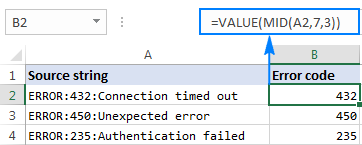The same approach works for more complex formulas as well. In the above example, assuming the error codes are of a variable length, you can extract them using the Mid formula that gets a substring between 2 delimiters, nested within the VALUE function:

`=VALUE(MID(A2,SEARCH(":",A2)+1,SEARCH(":",A2,SEARCH(":",A2)+1)-SEARCH(":",A2)-1))`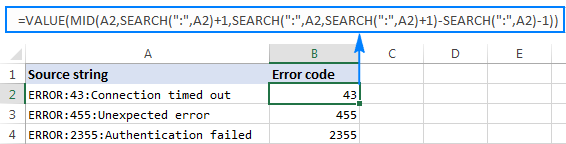This is how you use the MID function in Excel. To better understand the formulas discussed in this tutorial, you are welcome to download a sample workbook below. I thank you for reading and hope to see you on our blog next week!

Excel MID function - formula examples (.xlsx file)

## More examples of using the MID function in Excel:

1. Hi, I could not find the exact answer in my days finding, so trying to express below for a solution:
I need to extract and build a BP Number (Business Partner in SAP) from certain GL listing Text field. The problem is different users write the description in Text varyingly. The formula that I have built is giving me partial result as I mention below, the Row-6 is not giving the right pick :
Criteria:
1. Find "BP" in the Text.
2. Pick the number after BP up to the space.
3. Count the length of the number.
4. If the length is less than 10, then add 2000000000 to it, else if it is 10 then publish the same.

=IF(OR(C3="11479996", C3="11479997"), IF(LEN(TRIM(MID(N3,FIND("BP ",N3)+2,10)))<10, 2000000000+TRIM(MID(N3,FIND("BP ",N3)+2,10)),"NA"),"NA")

Text BP Number
Rej PY 6500272182 BP 10176376 PYT#4014644196 2010176376
BP#521636; PY#6500267772; PYT#4014644196 #VALUE!
Rej 6500284071 Index 657510 #VALUE!
REVERSAL 6500298182 Index# 794346 #VALUE!
Rej PY 6500302221 BP 10239350 no USD corresp bank 2010239350
Rej. PY 4015956978 BP 2010055678, accou. not exsit 2201005567

Would be grateful for the guidance.

Regards,
Javed

• Hi!

=IF(LEN((--MID(A1,SEARCH("BP",A1)+2,SEARCH(" ",A1,SEARCH("BP",A1)+3)-SEARCH("BP",A1)-1)))<10, (--MID(A1,SEARCH("BP",A1)+2,SEARCH(" ",A1,SEARCH("BP",A1)+3)-SEARCH("BP",A1)-1))+2000000000,(--MID(A1,SEARCH("BP",A1)+2, SEARCH(" ",A1,SEARCH("BP",A1)+3)-SEARCH("BP",A1)-1)))

2. I am trying to validate and bring some specific text to a cell using find function based on a key text. Below example for your reference:
Am-PE AN Type:Spouse;AM-P Count:Varies State;AM-P Design Cat:Hopefull, All Plats;OD_MR Call Value Instruct:Top Quiz
I tried this but didn't work =MID(A2,SEARCH("AM-P Count:",A2)+1,SEARCH(";",AY2)-SEARCH("AM-P Count:",AY2)-1).
I want to bring the value "Varies State" here. What I have suppose to do now

• Hi!
If I understand the problem correctly, to extract the text string try this formula:

=MID(A2,SEARCH("AM-P Count:",A2)+11, SEARCH(";",A2,(SEARCH("AM-P Count:",A2)+11)) - (SEARCH("AM-P Count:",A2)+11))

• It would be so helpful for me if you could help on the below too
*If header B1- Type(Am-PE AN Type:)
C1- Count(AM-P Count:)
D1- Cat(AM-P Design Cat:)
E1- Instruction(OD_MR Call Value Instruct:)

*A2 has the combination of texts:- Am-PE AN Type:Spouse;AM-P Count:Varies State;AM-P Design Cat:Hopefull, All Plats;OD_MR Call Value Instruct:Top Quiz
*B2 - I need to bring a set of text listed till ; from Column A2, which is if i need to bring "Spouse" in B2 what am I suppose to do,
also same for
C2 - "Varies States"
D2 - "Hopefull,All Plats"
E2 - "Top Quiz"

Additionally, the texts in A2 will be mixed every time

• If your values have the same pattern, you can use the new Excel functions TEXTSPLIT and CHOOSECOLS.
The formula for cell B2:

=CHOOSECOLS(TEXTSPLIT(A2,{",",";",":"}),2)

You can find the examples and detailed instructions here: TEXTSPLIT function in Excel: split text strings by delimiter and CHOOSECOLS function to get columns from array or range.

• The issue is am bringing the A2 value using a vlookup function from another sheet, so what happens here is it sometimes bring too many text strings swapped like below examples

Ex 1 - Fill with: Stories;Am-PE AN Type:Spouse;AM-P Count:Varies State;AM-P Design Cat:Hopefull, All Plats;OD_MR Call Value Instruct:Top Quiz;freq alert:2

Ex 2 - Am-PE AN Type:Spouse;AM-P Count:Varies State;AM-P Design Cat:Hopefull, All Plats;OD_MR Call Value Instruct:Top Quiz

So when use any formula with the key word "Am-PE AN Type:" in C2 cell it should only show the text string "Varies States" ending with ; and not the other texts like "Hopefull, All Plats" or "Top Quiz".

• Thank you so much sir. It helped a lot!

Cell = A2 - Name
B2 - Date
C2 - Date
D2 - formula cell

I want to validate the cell B2 and C2 which has dates and to find both are matching, I used =B2=C2 in cell D2 and if B2 & C2 matches it shows True if not it shows False.

And at the same time I want to use another formula in D2 to show the cell as Null if the A2 has no value. How do I show that? Anyhow B2 & C2 will have values only when A2 has value. so I need 2 formula's in D2. one is should should Null value when A2 is empty and True or false when B2 & C2 has value.

• Hi!
To check an empty cell A2, use the ISBLANK function. To check cells B2 and C2 by condition, use the IF function.
Try this formula:

=IF(ISBLANK(A2),"Null",B2=C2)

• Thank you so much Sir, it worked!!!

• Hi Sir, Sorry for coming back. Am facing another issue in validating the range of cells from A2 to C2.

The issue is I have A2= True; B2=True & C2=True, Now I want to validate the cell D2 which should show "Good" if all the 3 three cells A2,B2 & C2 has the value True.

And if any one of the cell(A2,B2 or C2) has False value the D2 should show Bad.

It will so helpful if you could help me in it.

3. Hello, i would like to provide the answer to question of Merc (february 14, 2023) about the way to extract the number/words with different length inside addresses.

This the formula to put in the first cell beside the address:
"=LEFT(A1;SEARCH(" ";A1)-1)"

This is the formula to put in the second cell beside the address:
"=MID(\$A1;COLUMN(B:B)+SUM(LEN(\$B1:B1));IFERROR(SEARCH(" ";\$A1;COLUMN(B:B)+SUM(LEN(\$B1:B1)))-SUM(LEN(\$B1:B1))-COLUMN(B:B);SUM(LEN(\$B1:B1))+COLUMN(A:A)))" ==> you can slide toward right

BR,
Nicolò

4. Hello! I'm hoping you can assist me with separating my address data, which I've provided examples of below. I would like to be able to separate the street number, street direction, and street name into separate columns. The issues I'm running into include different address lengths, some streets do not have a direction, and some street names are more multiple words. Thank you!

5020 N HIGH ST
5661 CRAWFORD DR
1425 CHELMSFORD SQ S
3535 W DUBLIN-GRANVILLE RD

• Hello!
Unfortunately, to use formulas or other tools to separate text by columns, all text strings must have the same pattern.

• Thank you for the quick response.

5. What is the answer to this question?

Cell A2 contains the US telephone number (939) 555-0113. What will be displayed using the formula =MID(A2,2,3)?

6. Hi,

Hi,
I have cell contains 2871 characters including special characters as given below : I am looking for each colon items in separate cell. I do not want to use text to column function but want to use formula using MID, right substitute,LEN etc. Please help.
Goods Description : VALVES, MANUAL 101-V3724 (PARTS OF MACHINERY)

Party Ref. No. : SA-USIN-OA4035 KIND ATTN :
Vessel : Invoice No. : PI10 - 2306675
:
POL : Invoice Dt. :
ETD :
Place of Supply :
POD : Gross Weight(Kgs) : 7336.000 Our Ref. : CISF-XXXXXXXXXXX-1
MBL/MAWB No. : XXXXXXXXXXXXXXXXX
Net Weight : 0
HBL/MAWB No. : No. Packages : 17
Volume : 7.336 Consignee Name :
IGM No. : 0 Shipper Name :
Item No. : Comm. Invoice Nos : EN-21-0919INV022,EN-21-0921INV027,EN-21-0922INV02
BOE No / Date : XXXXXX / 25-Oct-22 Total CIF Amt : 10982767.57 Total FOB Amt : 0.00

• Hi!
A formula with the functions you've specified is not possible with such a long text string. You can use the TEXTSPLIT function. See the description and examples in this article. Or use recommended tools like Split Text tool.

7. I have a set of data in column, in each string there are numbers and one letter only.
The letter can be in different position.
I need a formula to extract that letter.
Thanks

• Hello!
To extract only letters from a text string, you can use the custom REGEX function. Read the detailed description: Regex to extract strings in Excel (one or all matches).
Or try this formula:

=SUBSTITUTE((CONCAT(IF(NOT(ISNUMBER(--MID(A3,ROW(\$1:\$50),1))),MID(A3,ROW(\$1:\$50),1),"")))," ","")

8. I have a column from where I want to take out the grammage of that object. For example:

25.5 GRM 1881 Preform
2.74 GRM Closure
1.75 GRM Closures
10.46 GRM 29/25 Preform
PRE 7.2 GM CLEAR 29/25
PRE 7.2 GM BLUE 1 29/25 GC
PRE 7.8GM CLEAR 29/25 GC
PRE 7.8 GM BLUE 1 29/25 GC
PRE 7.8 GM BLUE 6 29/25 GC
19.0 GRM Preform

So, here first 04 can be easily extracted with Text to column and using space as delimited. I am facing issue where the text starts from PRE and also at one instant the GM is written without space.
I need a single formula to extract the grammage in numbers only that I can put in power query of excel table to work further.

This is getting very confusing.

9. Hi!

Intel(R) Core(TM) i7-1065G7 CPU @ 1.30GHz (8 CPUs), ~1.5GHz

How can I extract the "i7-1065G7"?

TIA!

• Hi!
Try this formula:

=MID(A1,19,10)

10. i have received data in a timer format and the requirement is to get the first response time
the data is in 16m,1h 31m,-194h 5m,-20m,3h, and the minus data represents the first response breached data and i have been using this formula which gave me negative value for few terms.
=iferror(left(J2,find("h",J2)-1)*60,0)+iferror(mid(J2,iferror(find("h",J2)+1,1),find("m",J2)-iferror(find("h",J2),0)-1),0)

• Hi!

11. Games Barney 20171027 \$1250

i want to extract date from above text. i have almost 500 row with same format but i couldn't figure out how to do this.
pls help me

• Hello!
We have a tool that can solve your task in a couple of clicks - Text to date tool. It is available as a part of our Ultimate Suite for Excel that you can install in a trial mode and check how it works for free.

• Thanks for replying. I definitely use this tool. For now i have an assignment where i need to use mid and find formula to extract date from text.
such as in this text : Games Barney 20171027 \$1250
i have to use Mid+find function to extract 20171027 from above text.

i hope i am able to convey my message.

12. How can I extract the characters between quotes when there are multiple instances of the delimiter and I am looking for a different result each successive column... IE:
"Animal":"Dog","Color":"Brown","Location":"New York City","Date":"January 2022"
the above text being in Cell A2, column headers B,C,D,E are Animal, Color, Location, Date respectively.
The position of the given result and the length of each response varies. I have had some luck getting the response pulled in, but cannot figure out how to account for the appropriate length of each unique response so some are truncated and others are pulling in more characters than needed... ie Brown","Lo

• For reference, this is what I had so far:
=MID(D2,FIND(H\$1,\$D2)+LEN(H1)+3,10), Where D2 is the source text and H1 is the category (column header)
The nested FIND formula is to determine the position where I would find the desired result in the source text.
Also, the \$ are inserted because I intend to carry it over columns and rows.

• Another variation trying to use the delimiters, but then I get stuck trying to incorporate the number of characters:
=MID(D2,FIND(H\$1,\$D2)+LEN(H1)+3,SEARCH("""",D2,SEARCH("""",D2)+1)-SEARCH("""",D2)-1)

• Hello!
To extract all strings between delimiters from text, use a user-defined function RegExpExtract.
You can find the examples and detailed instructions here: How to extract substrings in Excel using regular expressions (Regex)
Write the search pattern to a cell G2

'"[^"]+"

Use the formula:

=SUBSTITUTE(RegExpExtract(A2, \$G\$2),"""","")

To show the result by columns, use the TRANSPOSE function.

=TRANSPOSE(SUBSTITUTE(RegExpExtract(A2, \$G\$2),"""",""))

• Didn't work... returning blank results. I set the match_case to False just in case.
After a few more tweaks, I starting getting spill errors and it was pulling in the first couple characters into the first couple cells and then the remaining characters into a handful of cells below it.

13. Hi
It it possible to use the below formula to find multiple criteria? For example I am looking for letters beginning with GB and US but the current formula only returns those with GB.

=TRIM(MID(SUBSTITUTE(E151," ",REPT(" ",99)),MAX(1,FIND("GB",SUBSTITUTE(E151," ",REPT(" ",99)))-50),99))

• Hello!
The current formula cannot return "US". To do this, add the search "US". This can be done using the IFERROR function.

=IFERROR(TRIM(MID(SUBSTITUTE(E151," ",REPT(" ",99)),MAX(1,FIND("GB",SUBSTITUTE(E151," ",REPT(" ",99)))-50),99)), TRIM(MID(SUBSTITUTE(E151," ",REPT(" ",99)),MAX(1,FIND("US",SUBSTITUTE(E151," ",REPT(" ",99)))-50),99)))

14. This article gave me some perspective on how to build logic around these kinds of problems. With Google Sheets, you can just use the Split function and provide the delimiter and that does the job.

• Hello Daksh,

there are actually a lot more ways in Google Sheets to extract data from different parts of cells. Feel free to look through this article to get to know them.

15. A1 = Aaron (16 Years Old) [Gold Star]
A2= Peter (16 Years Old) [Sliver Star]
A3 = John (16 Years Old) [Bronze Star]

Hi, If i have the above in in A1, A2 and A3,

Can i use just one MID formula ti show
Gold in B1,
Silver in B2
Bronze in B3

Thanks in Advance for your help. i been trying and it is all error
Eugene.

• Hello!
To extract the word after the "[" character from the text, you can use the formula:

=MID(A1,SEARCH("[",A1)+1, SEARCH(" ",A1,SEARCH("[",A1)+1)-SEARCH("[",A1)-1)

Pay attention to the following paragraph of the article above - How to get substring between 2 delimiters.
SEARCH function finds the characters "[" and " ".
Then copy this formula down the column. You can also use autofill Excel formulas for this.

• Thank you Sir... Thank you i will have a look at the article again.

• Can you explain further the parts this formula please?

16. HI
with single statement can i pull the data.

1) FGH-0622_Disb2of4 (need to pull Disb2of)

2) DFG-0722_Disb20of40 (need to pull Disb20of)

the formula is correct for 1st MID(A5,SEARCH("Disb*f",A5),7)

• Hello!
You can't extract the second text using the MID and other substring functions because it has 8 characters instead of 7.
To extract text between characters, I recommend using regular expressions. You can find the examples and detailed instructions here: Regex to extract strings in Excel (one or all matches).

=RegExpExtract(A1,"\Disb.*?\of")

17. Hello

As shown below,i want to extract the number before /CAP for the first one
the second i want to extract the number before the /f.c

is it possible or not, If it is possible could you please let me know.

ROWACHOL 45/CAP 45 CAPS PKG
DAPAVELDACTIN PLUS 5MG/1000MG 28/F.C.TAB 28 STP PKG

18. ctrl+shift+enter

=TRIM(MID(SUBSTITUTE(CONCAT(IF(ISNUMBER(NUMBERVALUE(MID(SUBSTITUTE(\$A1,".",1),ROW(INDIRECT("1:"&LEN(\$A1))),1))),MID(\$A1,ROW(INDIRECT("1:"&LEN(\$A1))),1)," ")),". ",""),1,LEN(\$A1)))

aaaa111.2222bbbbb3333.4444ccccggggcc5555.66666ggffff=TRIM(MID(SUBSTITUTE(CONCAT(IF(ISNUMBER(NUMBERVALUE(MID(SUBSTITUTE(\$A1,".",1),ROW(INDIRECT("1:"&LEN(\$A1))),1))),MID(\$A1,ROW(INDIRECT("1:"&LEN(\$A1))),1)," ")),". ",""),1,LEN(\$A1)))

19. Dear Professor Alexander Trifuntov, it is a real pleasure to greet you.

Regarding the extraction of text from a cell I have a couple of doubts. I am testing some codes in VBA to obtain the RGB color range that Excel brings by default. The function (UDF) gives me the result in a cell (e.g. C2) in the form RGB(255,255,255).

I want to extract each of the corresponding values for each color in separate cells (e.g. D2:F2).

I have already managed to obtain the value for the color Red (R) that goes in cell D2 as follows =NUMBERVALUE(MID(LEFT(C2,FIND(",",C2)-1),FIND("(",C2)+1,LEN(C2)))

In the same way I have obtained the value for the color Green (G) that goes in cell E2 as follows =NUMBERVALUE(MID(C2,FIND(",",C2)+1,FIND(",",C2,FIND(",",C2)+1)-FIND(",",C2)-1))

However, I have not been able to get a formula to obtain the last value corresponding to the color Blue that would go in cell F2, (this would be approximately the values that are further to the right of the last comma).

Would you be so kind as to point me to a solution that would allow me to extract these last characters.
I clarify that each one of the values changes on the scale from 0 to 255, according to the corresponding color.

I appreciate your valuable help and attention to solve my doubt, thank you very much.

• Hello!

=MID(A2,FIND("(",A2)+1,FIND(",",A2)-FIND("(",A2)-1)
=MID(A2,FIND(",",A2)+1,FIND(",",A2,FIND(",",A2)+1)-FIND(",",A2)-1)
=MID(A2,FIND(",",A2,FIND(",",A2)+1)+1,FIND(")",A2)-FIND(",",A2,FIND(",",A2)+1)-1)

I hope this will help

• Dear Professor, thank you very much for your valuable collaboration, indeed, all the formulas work correctly, I appreciate your kind attention!

20. Hello,

Here is the string that I have - 'FINISHED GOODS:Ballistic Inserts:400 Series:4400:4400-SA-MC-M'

I'd like to extract '4400-SA-MC-M' from the end of it. I have a range of products that I'd like to extract that last string from but the string length (meaning number of characters in it) is not always the same (some part numbers are longer by one or two characters).

It would also be helpful to be able to extract the '400 series' and the '4400' separately.

• Hello!

=MID(A2,SEARCH("#",SUBSTITUTE(A2,":","#",LEN(A2)-LEN(SUBSTITUTE(A2,":",""))))+1,50)

21. 2-TMP-001B-212-AM-00006-01

I WANT TO EXTRACT 212-AM-00006
AS A RESULT, WHAT FORMULA SHOULD I HAVE APPLIED.

• =MID(A25,FIND("-",A25,FIND("-",A25,FIND("-",A25)+1)+1)+1,FIND("-",A25,FIND("-",A25,FIND("-",A25,FIND("-",A25,FIND("-",A25,FIND("-",A25)+1)+6)-FIND("-",A25,FIND("-",A25,FIND("-",A25)+2))))))

i used this formula, but the result is 212-DG-0000

Missing "5" at last, Result Must be 212-DG-00005

• Yes i understood your concern, but it has to be done more times again and again, could you tell me the formula.

• Hi!

22. I have on A1 and A2: In B1 and B2 I write: Resoult:
A1: HK\$11.720 +A2*3 #VALUE!
A1: HK\$0.127 +A2*3 #VALUE!

If I use:
For A1 (HK\$11.720) =(RIGHT(A2;LEN(A2)-FIND("\$";A2)))/1000 11,720 (as normal number) or: =(RIGHT(A2;LEN(A2)-FIND("H";A2)-FIND("K";A2)))/1000 11,720 (as normal number)

but if use the same formula for A2

For A2 (HK\$0.127) =(RIGHT(A2;LEN(A2)-FIND("\$";A2)))/1000 #VALUE! or: =(RIGHT(A2;LEN(A2)-FIND("H";A2)-FIND("K";A2)))/1000 #VALUE!

If I use (to add –FIND(“\$”;A2):

On A2 (HK\$0.12) =(RIGHT(A2;LEN(A2)-FIND(“H”;A2)-FIND("K";A2)-FIND("\$";A2)))/1000 0,027 (as normal number but, missing the “1”)

If I use:

On A2 (HK\$0.12) =(RIGHT(A2;LEN(A2)-FIND("K";A2)-FIND("\$";A2)))/1000 0,127 (as normal number)

Which formula can be use for the two quantities in one time?

Thanks

• Hi!
Unfortunately, I don't understand what you want to do.

• Sorry and thanks for your help, I try to clarify as:

I have:
A1.- HK\$11.720 Write in B1.- +A1*3 Resoult: #VALUE!
A2.- HK\$0.127 Write in B2.- +A2*3 Resoult: #VALUE!

If I use the formula on:
B1.- =(RIGHT(A2;LEN(A2)-FIND("\$";A2)))/1000 《or》
=(RIGHT(A2;LEN(A2)-FIND("H";A2)-FIND("K";A2)))/1000 Resoult: 11,720 (as normal number can use)

But (with the same formula for HK\$0.127 (decimal)), can not be use!
B2.- =(RIGHT(A2;LEN(A2)-FIND("\$";A2)))/1000 《or》
=(RIGHT(A2;LEN(A2)-FIND("H";A2)-FIND("K";A2)))/1000 Resoult: #VALUE!

If I use to add in B2: –FIND(“\$”;A2):
B2.- =(RIGHT(A2;LEN(A2)-FIND(“H”;A2)-FIND("K";A2)-FIND("\$";A2)))/1000 Resoult: 0,027 (as normal number but, missing the “1”)
If I cancel to use in B2: –FIND(“H”;A2) and add -FIND("\$";A2)
B2.- =(RIGHT(A2;LEN(A2)-FIND("K";A2)-FIND("\$";A2)))/1000 Resoult: 0,127 (as normal number can use)

NOTE:
A1.- HK\$11.720 should to be 11,720
A2.- HK\$0.127 should to be 0,127

Which formula can be to use for the two quantities HK\$11.720 to be 11,720 and HK\$0.127 to be 0,127 insted 2 formulas, one for natural number and one for decimal number?

I need to create an excel table with more than 2.500 datas/x column take (page/copy) from a Government HK web for financial companies in HK.

When I make the page/copy, the HK\$(+number) are no numeric and also I don´t know, ” its not as texto”, can not use on formulas, can not change from texto to number.

I can do/use it if I change/create as normal number without HK\$ and with “,” instead “.”, by create a formula as explain above.

Thanks Alexander

• Hello!
It is available as a part of our Ultimate Suite for Excel that you can install in a trial mode and check how it works for free.
You can replace "." with "," using the standard Find & Replace tool.

• A1.- HK\$11.720
A2.- HK\$0.127
A3.- HK\$0.048

Formula in B1 / B2 / B3: =(RIGHT(A2;LEN(A2)-FIND("\$";A2)))/1000 or =(RIGHT(A2;LEN(A2)-FIND("H";A2)-FIND("K";A2)))/1000

Resoult:
B1.- 11,720 (as normal number)
B2.- #VALUE!
B3.- #VALUE!

Formula in B1 / B2 / B3: =(RIGHT(A2;LEN(A2)-FIND(“H”;A2)-FIND("K";A2)-FIND("\$";A2)))/1000

Resoult:
B1.- 0,900 (as normal number but, missing the “11”)
B2.- 0,027 (as normal number but, missing the “1”)
B3.- 0,048 (as normal number)

Formula in B1 / B2 / B3: =(RIGHT(A2;LEN(A2)-FIND("K";A2)-FIND("\$";A2)))/1000

Resoult:
B1.- #VALUE!
B2.- 0,127 (as normal number )
B3.- 0,048 (as normal number)

• Hi!
To extract a number from the end of a text try this formula:

=--SUBSTITUTE(MID(A2,SEARCH("\$",A2)+1,50),".",",")

23. Hi, if I have a whole number like 20090206 and I want to format into dd:hh:mmss (20:09:02:06). How would I proceed to include the ":" delimiter spaced by two numbers? Thank you!

• Hi!
With the MID function, take every 2 digits from the number.

=MID(A1,1,2)&":"&MID(A1,3,2)&":"&MID(A1,5,2)&":"&MID(A1,7,2)

The formula returns text.
You can use a custom number format:

##":"##":"##":"##

24. In a single cell I have a cluster of numbers separated by tabs or spaces ; I am trying to isolate just the starts with 79 numbers that are always 13 digits in a separate cell
Example...
7981107135154
110567815X
1316135086

I was using formula =TRIM(LEFT(SUBSTITUTE(MID(C5,FIND("7",C5),LEN(C5)),"",REPT("",LEN(C5))),LEN(C5))) but it doesn't put a stopper to the digits so I get everything that follows it.

Any Ideas how I can isolate it?
Thanks
NEMA

• Hi!
Sorry, it's not quite clear what you are trying to achieve. Explain in more detail. Give an example of your cluster of numbers and the desired result.

25. Thank you for the formula. It works.

26. Hi,

I am trying to convert 30-jun-21 01.45.18.488173000 pm to 1:45. Please help someone with mid and substitute function together.

• Hello!

=TIME(MID(A2,SEARCH(" ",A2,1)+1,2),MID(A2,SEARCH(" ",A2,1)+4,2),0)

• Can I get the time in 1:45 PM? The formula works but it shows 1:45 AM.
Please advise on how timestamp can be shown in both AM and PM when pulled the respective data in AM and PM.

• Hi. The above formula works to convert into normal time format.

The above timestamp format is 30-jun-21 01.45.18.488173000pm which is 1:45:00.

I have got four timestamps:
17:39:38
17:41:35
17:45:35
17:45:00( convention of above mentioned timestamp 1:45:00 +TIME(4,0,0)

I am trying to get the time difference with consecutive timestamps which I will get 3 time difference.

Problem: I have applied ABS(timestamp1-timestamp2) to find each difference.

But when I apply, =MAX(timedifference1,timedifference2, timedifference3)

I get the time difference of last two timestamps which is of 35 secs as maximum value. And I tried to convert all to text that shows the last timestamp is taking both date and time in calculation.

And other two difference are 00:01:57 and 00:04:00.

I should get 00:04:00 as maximum value. Please help me out on this as I am trying with random formulas but no success.

• Hi!
Using your formulas, I got the maximum value 00:04:00.

• Hi,

I am trying with the formula TIME(MID(A2,SEARCH(“ “,A2,1)+1,2),MID(A2,SEARCH(“ “,A2,1)+4,2),0).

The timestamp is showing as 12:07:00 PM.
I am not getting the “ss” number as result.
It should be 12:07:24.

• Hi. I was applying the formula in a rough data timestamps. When I apply it on real data sheet timestamp. The file is pulled from a sql, it is not capturing the ‘ss’ value.

27. This is awesome! I am managing an excel sheet of my expenses and I just copy pasted your formula =MID(A2,SEARCH(", ",A2)+1,SEARCH(", ",A2,SEARCH(", ",A2)+1)-SEARCH(", ",A2)-1)

It was so accurate. Thank you for sharing this! Amazing!

28. caution, this webeditor corrupts the formulas when posting. I did type a completely different formula than the one stated in my comment above!!!!! don't use it!

29. the string published above for selecting a string of letters between certain keys in that string is unfortunately wrong. The function MID requires more brackets, and uses semicolon.
Use this one if you want to select the string between "". E.g. in field A2 you have the string: improfessionalexcel, which will give you the word "using".

=MID(A2;SEARCH("";A2)-(SEARCH("<";A2)+1)))

• Hi!
The formula is correct and works. The use of semicolons depends on the regional settings of your Windows.

30. Hello! I hope you guys can help me with my case :)

%B6009210202107390^SMITH/JOHN I ^4912120000004825853 000 ?;6009210202107390=49121200000000000000?

If I would want to extract "^4912120000004825853" how should I use this formula?

Thanks!

• Hello!
If I understand your task correctly, the following formula should work for you:

=MID(A2,SEARCH(" ^",A2,1)+2, SEARCH(" ",A2,SEARCH(" ^",A2,1)+1) - (SEARCH(" ^",A2,1))-2)

Hope this is what you need.

31. I need the get the following characters

FG-F19951-D02-12 = get the new colomn D02

• Hello!
You can use this formula:

=MID(A2,11,3)

If this is not what you wanted, please describe the problem in more detail.

32. My work consists of string with two occurrences of this sign ":" , i.e. Account Num.:76372/B098:COM and I want to get only 76372/B098 in a single column.

I found the formula MID(A2,SEARCH(":",A2)+1,SEARCH(":",A2,SEARCH(":",A2)+1)-SEARCH(":",A2)-1) is very helpful and exactly solved my problems.

Thank you so much. You really help my work more easily. :)

33. hello,
i hneed a little help
i have a excel with some documents that have barcodes and i time stamp when are they created and i need to do somehow so that i know how many unfinicheddocuments i have before 14:00.the format of the cell is like this 08.03.2021 14:36:45,it is from excel extracted from e program that we use.i tried this formula : MID(D3;12;2)+IF(H3<14;"overdue";"still in frame") but is not working,separatly they are working but i tried to have just one formula.i think the error is from H3 from IF function.tks

34. Hello,

I want to know that how to extract any text from the cell.
There can be some sentences.

Thank You...

• Hi,
Have you tried the ways described in this blog post? If they don’t work for you, then please describe your task in detail, I’ll try to suggest a solution.

35. Hi,

I have 100s of rows of entries with different time stamps similar to -
2021-01-25T12:32:56.698+0000

These time stamps range for a period of up to 30 days, from 8am - 11pm
I've used LEFT and MID to create 2 new columns with date and time, such as 2021-01-25 & 12:32:56

So I now have 100s of entries with a nice date - time format

Now I want to get a total of how many times there's an entry recorded between 08:00-12:00 - 12:-01-16:00 - 16:01-20:00 and 20:01-23:00
,
I can't work out how to do this, as you can't sum the results, as they are output from the LEFT/MID queries.

• Hello!
Your date and time are recorded as text. Use the DATEVALUE and TIMEVALUE functions to convert them.
Here is the article that may be helpful to you: Using Excel COUNTIF function with dates.
To calculate the time, you can use something like this formula

=COUNTIF(B1:B4,">"&TIME(19,0,0))

36. Hi,
So I have thousands of SKU numbers with design, width, and length code included. I want to break the code down as I wish. I can use the MID function but not all the codes have the same character number or sequence.
Some examples of the SKUs:
0101_27in02ft
CUS-1980-32in07ft
STD8702-8X10

As you can see above first design code comes, then width and length.
How can I break down approx. 12000 items in my list?
Your help will be greatly appreciated.

Happy new year,
Tim

37. IMPS/P2A/036310898577/9485/8812103889

can anyone help me find 036310898577 this one

38. Choice hotel | US performance report | WC 18 Dec | 24 Dec | 1700 est

How i can split this by using formula in excel,
As required choice hotel in different column, US performance report in different column, and 24 Dec and 1700 est in different column.

39. Hi,

How do I extract the LAST word from the cell that contains any of the specific characters?

Example:
1. FirstName LastName 7.8 2
2. FirstName LastName 7.9 2
3. FirstName LastName 8.1 7.5 3
4. FirstName LastName 1:04.84
5. FirstName LastName 1:15.84 1:07.84

Should return:
7.8
7.9
7.5
1:04.84
1:07.84

Thanks!

• Hello!
Pay attention to the following paragraph of the article above - How to get the last name.
1:04.84 - last word
7.8 - not last word.

40. Awsome work Alexander. Works perfectly!

41. Hello

I use the following formula =-LOOKUP(1,-LEFT(MID(A1,MIN(SEARCH({0,1,2,3,4,5,6,7,8,9},A1&"0123456789")),LEN(A1)-MIN(SEARCH({0,1,2,3,4,5,6,7,8,9},A1&"0123456789"))+1),ROW(INDIRECT("1:"&LEN(MID(A1,MIN(SEARCH({0,1,2,3,4,5,6,7,8,9},A1&"0123456789")),LEN(A1)-MIN(SEARCH({0,1,2,3,4,5,6,7,8,9},A1&"0123456789"))+1))))))

This gives me the first set of digits (202009260630) in the string below, however I need to change this around so it gives me the last set of digits between underscore and .zip, in this case (1). I hope its just some minor adjustments to the provided formula that is needed.

If anyone is thinking of more smooth solutions I can add that the amount of underscores varies, and that the amount of digits between last underscore and.zip also varies. IE string length varies

Ramen_files_202009260630_1.zip

• Hello!
Please describe your problem in more detail. Include an example of the source data and the result you want to get. It’ll help me understand your request better and find a solution for you.

• So Sourcedata look like this;
gateway_files_202009250630_21.zip
Big_files_202009300630_2.zip
Glimmering_dust_stars_202010010630_5621.zip

The formula i provided in the first post provides me with the following set of digits from each row;
202009250630
202009300630
202010010630

Now, what I wan't it to do is to provide me with the last set of digits in each string;
21
2
5621

Let me know if you need me to elaborate further :)

• Hello!
The question of how to extract all the numbers from the text has been asked many times. Here is one of the answers.

• Hello Alexander,

I will elaborate further. Regarding the link you sent me, none of the examples include a string with 2 different set of digits(numbers in them). I'm not interested in extracting all numbers. I am interested in extracting the last set of numbers that in this case is between the last _ and .zip

The link also mentions using positions, but position varies as the filename lenght varies.

The reason why I provided this formula: =-LOOKUP(1,-LEFT(MID(A1,MIN(SEARCH({0,1,2,3,4,5,6,7,8,9},A1&"0123456789")),LEN(A1)-MIN(SEARCH({0,1,2,3,4,5,6,7,8,9},A1&"0123456789"))+1),ROW(INDIRECT("1:"&LEN(MID(A1,MIN(SEARCH({0,1,2,3,4,5,6,7,8,9},A1&"0123456789")),LEN(A1)-MIN(SEARCH({0,1,2,3,4,5,6,7,8,9},A1&"0123456789"))+1))))))

Is because the way I understand it is that it searches from the left til it hits the first digit(number) and continues until it hits a non number Thus providing me with the whole numberseriers of only the first continous numberseries.

I'm no expert in this but I thought maybe one of the experts here knew how the formula i provided could be tweaked to do exactly the same, but start from the right, so I get the last continuous numberseries of the source data.

In other words I want the formula to return 21 from the following source data; gateway_files_202009250630_21.zip

• Hello!
Here is the formula that should work perfectly for you:

=MID(SUBSTITUTE(A2,"_","@",LEN(A2)-LEN(SUBSTITUTE(A2,"_",""))), FIND("@",SUBSTITUTE(A2,"_","@",LEN(A2)-LEN(SUBSTITUTE(A2,"_",""))))+1, SEARCH(".",C8,1)-SEARCH("@*.",C8,1)-1)

Hope this is what you need.

• Hello Alexander,

Im not an expert in this, but it seems like you refer to something in cell C8, It's not obvious to me what this is. I assume the filename is located in A2.

Are you able to provide a picture of the extracted result from the file with the sourcedata where the formula is applied? :)

• Hello,
I am sorry for the inaccuracy. Here is the complete formula

=MID(SUBSTITUTE(A2,"_","@",LEN(A2)-LEN(SUBSTITUTE(A2,"_",""))), FIND("@",SUBSTITUTE(A2,"_","@",LEN(A2)-LEN(SUBSTITUTE(A2,"_",""))))+1, SEARCH(".",SUBSTITUTE(A2,"_","@",LEN(A2)-LEN(SUBSTITUTE(A2,"_",""))),1) -SEARCH("@*.",SUBSTITUTE(A2,"_","@",LEN(A2)- LEN(SUBSTITUTE(A2,"_",""))),1)-1)

42. I have an excel with transaction downloads from my bank. One column has the entries for the vendor of teh transaction but all are long and can differ. I want to extract one word (the vendor name) and place it in the next column in that row.

So, let's say that the cell D48 has a long string such as:
"DEBIT PURCHASE RET - VISA AMAZON.COM AMZN.AMZN.COM/BILWA".
I want to find one word AMAZON and place that in cell E48.

I will use the formula you provide to do this function for all my vendors, like PGE, AT&T, "Fred Meyer"

• Hello!
The information presented to you is not enough to give you advice.
What pattern do you want to search for a supplier's name?
Give some examples of the source data and the expected result.
It’ll help me understand it better and find a solution for you.

43. Hi
I would need some help on following extraction:
I need to extract the sender person of a bank transaction. So, I need to extract the words between the IBAN (which always starts with CH* and always has 21 digits) and between the word SENDER. IBAN is variable and count of extracted words as well (2,3 or 4 depending on sender reference name).
Example of my cell:
GIRO BANK CH9909990456345323499 Tester Max Noah Bern SENDER REFERENCE
Extraction should be "Tester Max Noah Bern"
Thanks a lot!

• Hello!
If I understand your task correctly, the following formula should work for you:

=MID(E1,FIND("CH",E1,1)+22,FIND("SENDER",E1,1)-(FIND("CH",E1,1)+22))

We have a tool that can solve your task in a couple of clicks: Ablebits Data - Split Text.

This tool is available as a part of our Ultimate Suite for Excel that you can install in a trial mode and use for free: https://www.ablebits.com/files/get.php?addin=xl-suite&f=free-trial

Unfortunately this does not work, because we have 2 problems:
- leading words can be different in count and variation (can have 4,6,7.. words)
- and leading words can include "CH" as well
e.g. another row looks like this:
GIRO BANK RAIFFEISEN SCHWEIZ GENOSSENSCHAFT DORFSTRASSE 99 999999 ALIGDNSLIW CH9812349000003109099 Beatrice Tester Bernstrasse 99 1234 Bern SENDER
From here I would have to extract "Selina"" ""Howald"" "Bern".
So I always have to extract the 2 words after the IBAN (right from CH*) and 1 word left from "SENDER". Count of words at beginning can differ as written above.

• Hi
Thanks, I tried your tool (Mac version) and it would work as workaround.
But still I would be looking more in a formula, because this formula will be part of a longer formula in which I consider different types of extractions.
So, how would I split text with mask by formula? Or how would I create the formula for my need described above?

44. I have a problem like this, the more number of letters I will separate, for example G: \ DCC \ EPC \ PIPELINE \ MRPR \ FINAL DOCUMENTATION
I want to separate 1 (G), 2 (DCC), 3 (EPC), 4 (PIPELINE), 5 (MRPR), 6 (FINAL DOCUMENTATION) please find a solution, Thank you very much

• Hello!
I recommend using the article on how to split text in a cell into columns.

• but if possible I need a formula for my problem, Thanks

• G:\DCC\EPC\PIPELINE\MRPR\FINAL DOCUMENTATION
G, I Use Formula =MID(A2,1,SEARCH(":\",A2)-1)
DCC, I Use Formula =MID(A2,SEARCH("\",A2)+1,SEARCH("\",A2,SEARCH("\",A2)+1)-SEARCH("\",A2)-1)
EPC, I don't know yet, solution please
PIPELINE, I don't know yet, solution please
MRPR, I don't know yet, solution please
FINAL DOCUMENTATION, I don't know yet, solution please
Thanks You Very Much

• Hello!
Your text uses the same "\" separators. Therefore, I recommend using the Excel tool "Text to columns".

• Thank you, I understand what you mean, it's easier to use the excel data facility "Data -> Text to Columns -> chose delimited -> next -> others -> \ -> next -> finish" but I ask for a solution with the formula if possible, Thank you very much
Hairul Alam

45. How to search three values using mid function i.e. use of "OR" statement in mid formula so we can able to search specific value thats meets two criteria (i have a lot of excel rows containing data in which sonme of them have personal information of persons and some of them are containing word "Vacant" so i would try to extract data of all cell and result shown personal data of persons and as well as word "vacant" shown if the cell containing data "Vacant". please help me!!!

46. I am trying to extract the last characters in a string. However the string is not always the same length nor the number of characters I need to extract, example:
29374sp
aw825885hxpc
ae4528-10xp
I need the characters at the end of the string after the numbers. Any help would be great.
Thanks

• Hello!
To get all characters in the text after the last number, you can use the formula

=RIGHT(A2,LEN(A2)-MAX(IFERROR(IF(SEARCH({1,2,3,4,5,6,7,8,9,0},MID(A2,ROW(\$1:\$99),1))=1,ROW(\$1:\$99)),0)))

I hope this will help

47. Hello guys, can someone help with getting the first value on a text.
So i want to get the 1st numbers on this text:
ABCDEFG 1234 (ABCD 1920) I want to get the 1234
BCBAKHJFAKFAHKLJS 1237214 (BABY-HKLJ) I want to get the 1237

• Hello!
To extract the first 4 digits from the text, you can use this formula

=MID(A1,MATCH(0,--ISERROR(-MID(A1,ROW(\$1:\$99),1)),),4)

Hope this is what you need.

• It works like charm! Thank you so much Alex!

• I'm working on a large amount of data. What i want is to determine if the old plan versus the new plan is a "Upgrade", "Downgrade" or "Retain".

Sample: Old Plan vs New Plan
Family Plan 1299 vs FamCombo 1699 result "Upgrade"
ValuePack 599 (Data 299) vs Family Plan 1999 (All in 1299) result "Upgrade"
So the bases plan is the 1st number. How can i construct the IF statement on this one? Need your help!

Thank you so much!

• Hello Suanson!
I’m sorry but your task is not entirely clear to me. Could you please describe it in more detail?
Why "Family Plan 1299 vs FamCombo 1699 result" Upgrade "? Under what condition will the result be "Downgrade" or "Retain"?
Is this entry in one cell or in several?

• It's like this...
If B is greater than A then it will display "Upgrade"
If B is less than A then it's a "Downgrade"
IF B is equal to A then the plan has been retained "Retain"
(B is the old plan while A is the new plan)

• Hello!
You haven't answered all my questions. I don't want to waste time guessing. I ask again. "Family Plan 1299 vs FamCombo 1699" - written in one cell or in several? Are the numbers 1299 and 1699 being compared? If you want help, please describe in detail your problem.

• It's written in different cell.
Column A Family Plan 1299
Colum B FamCombo 1699

and yes, the numbers are being compared. Sorry i have a very limited time in the office, and can only access it here.

48. (Backlash X @ -3.5 @ 4.0mm) If I want extract the value between @ and @ in the next cell @ and mm how to apply the mid and find function...

• Hello!
The formula below will do the trick for you:

=MID(A1,FIND("@",A1,1)+1,FIND("@",A1,FIND("@",A1,1)+1)-FIND("@",A1,1)-1)

I hope this will help

49. How can i extract the first number on these data.

ThePLAN PLUS 1299 (Value 1899)
ThePLAN 1799
ThePLAN PLUS 1299 (Value 1899)

• Hello!

=--MID(SUBSTITUTE(B2," ",""),MATCH(TRUE,ISNUMBER(--MID(SUBSTITUTE(B2," ",""),ROW(\$1:\$24),4)),0),4)

I hope this will help

• Thank you so much Sir Alexander Trifuntov (Ablebits.com Team), you're awesome!!!

50. could someone help to get the output only with the date. There are 2 different dates in one column..
Generate on Begin time :Sun Jul 5 0:0:0 2020
GenerateOn Begin time : Sun May 10 05:36:52 2020
I would require the output as MM/DD/YYYY Only from both the fields..

• Hello!